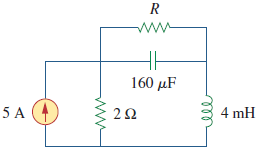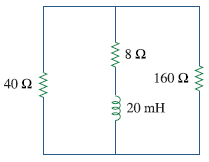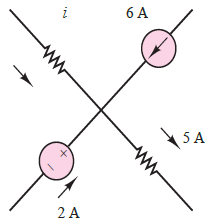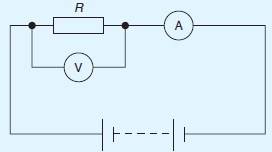# SSC JE Electrical Important MCQ Part 22

SSC JE Electrical Important MCQ PDF Part 22

1.For the circuit in Fig,What is the value of R that will make the energy stored in the capacitor the same as that stored in the inductor under dc conditionsA. 5Ω

B. 10Ω

C. 15Ω

D. 20Ω

(Under dc conditions, Capacitor-open circuit,Inductor-Short circuit so equivalent circuit is as belowiL=2×5/(R+2)=10/(R+2)

vc=RiL=10R/(R+2)

Energy Stored In Capacitor Wc=1/2(Cvc²)=80×10⁻⁶×100R²/(R+2)²

Energy Stored In Inductor WL=1/2(LiL²)=2×10⁻³×100/(R+2)²

As per condition Wc=WL

80×10⁻⁶×100R²/(R+2)²=2×10⁻³×100/(R+2)²

80×10⁻³×R²=2

R=√2/80×10⁻³=√1000/40=√25=5 Ω)

2.What is the time constant for the RC circuit in FigA. 20 ms

B. 30 ms

C. 15 ms

D. 10 ms

(τ=Rth×C,Rth=Thevenin equivalent at the capacitor terminals

So Rth = 120 || 80 +12 =(120×80/200)+12=48+12=60 Ω

τ=60×0.5×10⁻³=30 ms)

3.What is the time constant for the RL circuit in FigA. 0.3 ms

B. 1.5 ms

C. 0.5 ms

D. 1.0 ms

(τ=L/Rth

Rth=Thevenin equivalent at the inductor terminals

So Rth = 40 || 160 +8 =(40×160/200)+8=32+8=40 Ω

τ=(20×10⁻³)/40=0.5 ms)

4.What is the current I in the circuit of FigureA. -3 A

B. 3 A

C. 5 A

D. -5 A

Answer : A (I+2+6=5 or I=5-8=-3 A)

5.If in the circuit shown in Fig.the reading on the voltmeter is 5V and the reading on the ammeter is 25 mA, the resistance of resistor R isA. 0.005Ω

B. 5Ω

C. 125Ω

D. 200Ω

6.The capacitance of a capacitor is the ratio

A. charge to p.d. between plates

B. p.d. between plates to plate spacing

C. p.d. between plates to thickness of dielectric

D. p.d. between plates to charge

7.A magnetic pole face has a rectangular section having dimensions 200 mm by 100 mm. If the total flux emerging from the pole is 150 μWb,Then the flux density is

A. 7.5T

B. 0.0075 mT

C. 7.5 mT

D. 0.5 mT

(Flux =150 μWb=150×10⁻⁶ Wb

Cross sectional area A=200×100=20000 mm²=20000×10⁻⁶ m²

Flux density, B = φ/A=150×10⁻⁶/20000×10⁻⁶ = 0.0075T or 7.5mT)

8.What is the value of reluctance of a piece of mumetal of length 150 mm and cross-sectional area 1800 mm² when the relative permeability is 4000.

A. 16580/H

B. 16.580/H

C. 165.80/H

D. 1658/H

(S = l/μ₀μᵣA= 150 × 10⁻³/(4π × 10⁻⁷)(4000)(1800 × 10⁻⁶)= 16580/H)

9.The effect of an air gap in a magnetic circuit is to

A. increase the reluctance

B. reduce the flux density

C. divide the flux

D. reduce the magnetomotive force

(Reluctance (S) is the ‘magnetic resistance’ of a magnetic circuit to the presence of magnetic flux.The air gap in magnetic circuit means the magnetic resistance, i.e. reluctance to be increase)

10.A relay can be used to

A. decrease the current in a circuit

B. control a circuit more readily

C. increase the current in a circuit

D. control a circuit from a distance

11.There is a force of attraction between two current-carrying conductors when the current in them is

A. in opposite directions

B. in the same direction

C. of different magnitude

D. of the same magnitude

12.The frequency of the supply to the stator of an 8-pole induction motor is 50 Hz and the rotor frequency is 3 Hz. Value of the slip will be

A. 6%

B. 0.6%

C. 60%

D. 0.06%

Answer : A (fr =sf . Hence 3=(s)(50) or slip, s = 3/50= 0.06 or 6%)

13.The symbol for the unit of temperature coefficient of resistance is

A. Ω/⁰C

B. Ω

C. ⁰C

D. Ω/Ω⁰C

Answer : D (The SI unit of temperature coefficient of resistance is /⁰C )

14.The greater the internal resistance of a cell

A. the greater the terminal p.d.

B. the less the e.m.f.

C. the greater the e.m.f.

D. the less the terminal p.d.

Answer : D (Terminal p.d.=V = E − Ir, Where r is Internal resistance)

15.Figure shows three lamps, each rated at 240V,connected in series across a 240V supply.If another lamp of similar rating is added in series with the other three lamps thenA. each now glows even more dimly

B. each now glows even more brightly

C. lamps will not light up

D. lamps are unaffected

Answer : A (If another lamp of similar rating is added in series with the other three lamps then each lamp now has (240/4)V, i.e. 60 V across it and each now glows even more dimly)

16.With the switch in Fig. closed, the ammeter reading will indicateA. 1A

B. 75A

C. 1/3 A

D. 3A

17.Two parallel rectangular plates measuring 20 cm by 40 cm carry an electric charge of 0.2 μC.The electric flux density will be

A. 2.5μC/m²

B. 25μC/m²

C. 250μC/m²

D. 0.25μC/m²

(Area=20 cm×40 cm=800 cm² =800×10⁻⁴ m² and charge Q=0.2 μC=0.2×10⁻⁶ C

Electric flux density D = Q/A=0.2×10⁻⁶ /800×10⁻⁴=(2000/800)×10⁻⁶=2.5μC/m²)

18.Which of the following would apply to a moving coil instrument

A. An uneven scale, measuring d.c.

B. An even scale, measuring a.c.

C. An uneven scale, measuring a.c.

D. An even scale, measuring d.c.

Answer : D ( Moving coil instrument is used to measure DC and its scale is linear or even)

19.A potentiometer is used to

A. compare voltages

B. measure power factor

C. compare currents

D. measure phase sequence

Answer : A (A potentiometer is an instrument for measuring voltage or ‘potential difference’ by comparison of an unknown voltage with a known reference voltage.)

20.When a silicon p-n junction diode is reverse biased

A. a constant current flows over a large range of voltages

B. current flow is due to electrons in the n-type material

C. the magnitude of the reverse current flow is usually less than 1μA

D. none of these

Answer : B (Free electrons (minority) charge carriers carry the electric current in reverse biased p-n junction diode)